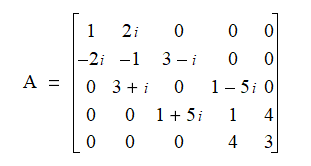# Z-paper-435 --> Square matrices

This Z-paper 435 by Herb Zinser   uses Nature's SYMBOL MACHINE words, languages, proper nouns, equations, and concepts to understand the CRYPTIC writings of newspaper reporters, editors and publishers,  and book authors.

The SYMBOL MACHINE is comprised of English language words and concepts, math and physics equations, biochemistry diagrams, electromagnetic sin wave schematics, etc.   The SYMBOL MACHINE equivalent are basic college textbooks in math and science  that may be found in any  bookstore  or a bookstore near a university.

We are reminded of ......

Language in thought and action - S. I. Hayakawa - Google ...

books.google.com › Language Arts & Disciplines › Linguistics › General‎

A revised, updated edition of S. I. Hayakawa's classic work on semantics. He discusses the role of language, its many functions, and how language shapes our ... .... Semantics is primarily concerned with meaning and reference, i.e. what Hayakawa calls the relationship between the «map» and the «territory» ...

Maps and Territories - Rijnlandmodel

Language in Thought and Action, S.I. Hayakawa.
Chapter 2 Symbols Maps and Territories
There is a sense in which we all live in two worlds.

Thus we have the source domain of SYMBOL LIFE and thought that may get mapped to the destination range of physical biology with human activities of the geography surface of EARTH.Let's look at some proper nouns and other words that provide CLUES to Nature's message processing system ... with possible messages for  people that are interested in CRYPTIC situations.

Hermitian matrix - Wikipedia, the free encyclopedia

en.wikipedia.org/wiki/Hermitian_matrix‎

Wikipedia

In mathematics, a Hermitian matrix (or self-adjoint matrix) is a square matrix with complex entries that is equal to its own conjugate transpose—that is, the ...

15.8 Hermitian Banded Matrices (.NET, C#, CSharp, VB, Visual Basic, F#)

A Hermitian banded matrix is a Hermitian matrix (Section 15.4) that has all its non-zero entries near the diagonal. Entries farther away from the diagonal than the half bandwidth are defined to be zero. That is, if is the half bandwidth, then whenever or . For example, this is a 5 x 5 Hermitian banded matrix with a half bandwidth of 1:Below, we see the Darwinian SYMBOL evolution of blood --> alpha/numeric blood -->   B  + number code 100  + D

binary number code  --> 100 --> decimal number 4  LINK to 4 DNA nucleotides of thought

decimal number code --> 100 --> 10 fingers times 10 toes = one hundred --> decimal accounting systems

Hex'100' = level 256 decimal ---> level 256 b100D --> the hierarchy problem in human bio-physics MESSAGE recognition systems

Thus we see some pieces to several puzzles about Nature's systems

Write a comment